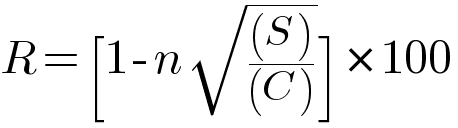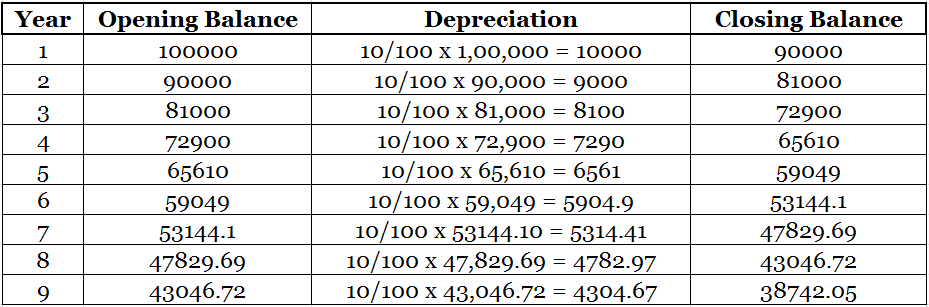# How To Calculate Scrap Value of an Asset?

## Calculate Scrap Value of an Asset -SLM/WDV

The scrap value of an asset may be defined as the maximum value that can be fetched by salvaging or selling it after its useful life. It is also known as salvage value, residual value or break-up value.

To know how to calculate the scrap value of an asset it is important to remember the below formula## How to Calculate Scrap Value of an Asset with SLM Depreciation

Straight Line Method Formula = (Cost of Asset – Scrap Value)/Useful Life in Years

Unreal Corp. Pvt Ltd. purchases machinery worth 1,00,000

Cost of machine = 1,00,000 | Estimated life of the asset = 9 years | Depreciation (Straight Line Method) = 10% p.a

Depreciation year 1 = 10/100 * 1,00,000 = 10,000

Cost of asset after Year 1 = 1,00,000 – 10,000 = 90,000

Similarly total depreciation for 9 years = 10,000*9 = 90,000

To Calculate Scrap Value of an Asset = Cost of Asset –  Total Depreciation

After 9 years scrap value  = 1,00,000 – 90,000 = 10,000

Related Topic – More Assets Related Questions and Answers

## How to Calculate Scrap Value of an Asset with WDV Depreciation

Written Down or Diminishing Balance Method

Written Down Value Method Formula =Using the same example as above, Unreal Corp. Pvt Ltd. purchases machinery worth 1,00,000

Cost of machine = 1,00,000 | Estimated life of the asset = 9 years | Depreciation (Written Down Value) = 10% p.a

The same formula is used to calculate the scrap value of an asset whichever method of depreciation is used (SLM/WDV)Scrap Value= 100000 – 61257.95 = 38742.05

The above examples should make it easy for anyone aspiring to learn how to calculate the scrap value of an asset, especially with the help of some examples.